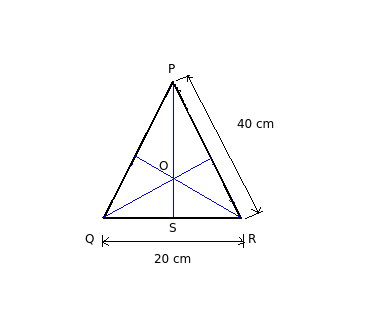# Center of gravity - Isosceles triangle

Q.  What is the C.G of an isosceles triangle of base 20 cm and side 40?
- Published on 18 Sep 15

a. 12.90 cm
b. 13.28 cm
c. 19.36 cm
d. 38.72 cm

#### Discussion

• Punit kumar singh   -Posted on 14 Oct 19
Feom where base, ya top if we take from base then it will right but if u take from top it will be 2h/3
• Sravanthi   -Posted on 14 Dec 15
Given: Base of an isosceles triangle = 20 cm, side = 40 cm

Formula: Centroid = Height of isosceles triangle (h) / 3

Solution:- O is the centroid of triangle PQR.

- Height of triangle = √(40)2 - (10)2

= 38.72 cm

Centroid of triangle along y – axis = h / 3

= 38.72 / 3

= 12.90 cm

## ➨ Post your comment / Share knowledgeEnter the code shown above:

(Note: If you cannot read the numbers in the above image, reload the page to generate a new one.)